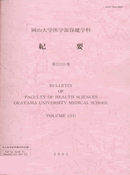Bulletin of Faculty of Health Sciences Okayama University Medical School
Published by Faculty of Health Sciences Okayama University Medical School

<Formerly known as>

数学と数学教育

With the 17-th century an essentially new period in the development of mathematics began. The circle of quantitative relations and spatial forms of mathematics studied now was no longer exhausted by numbers, quantities and geometric figures. On this basis there resulted the explicit introduction into mathematics of the ideas of motions and change. Being inseparably connected with the needs of natural science, the accumulation of quantitaive relations and spacial forms studied in mathematics was continuously expanding. However, in addition to this quantitative grouth, at the end of the 18-th and the beginning of the 19-th centuries a number of essentially new features were observed in the development of mathematics. The enormous amount of factual material which had been accumulated in the 17-th and 18-th centuries led to the demand for a deep logical analysis and unification of it from a new point of view. In essence, the relationship between mathematics and natural science was no loss close but was now increased in complexity. The majority of new theories arose from internal requirements of mathematics itself. However, the circle of applications to problems of science and technology was greately expanding at this time. In this way, as a result of both the internal requirements of mathematics and the new needs of science and technology, the circle of quantitative relations and spatial forms studied in mathematics was greately expanded: relations between elements of arbitrary groups, operations in function spaces, etc. are now parts of mathematics. In this paper, we are concerned with mathematical thought. Several ideas of great scientists are introduced, and we know the essence of mathematical thought. The power of mathematical thought can be brought up by means of a deep thought. The relationship between mathematical thought and creativity is also studied.
キーワード
Mathematics
Mathematics Education
Mathematical Thought
Creativity
ISSN
0917-4494
NCID
AN10355371